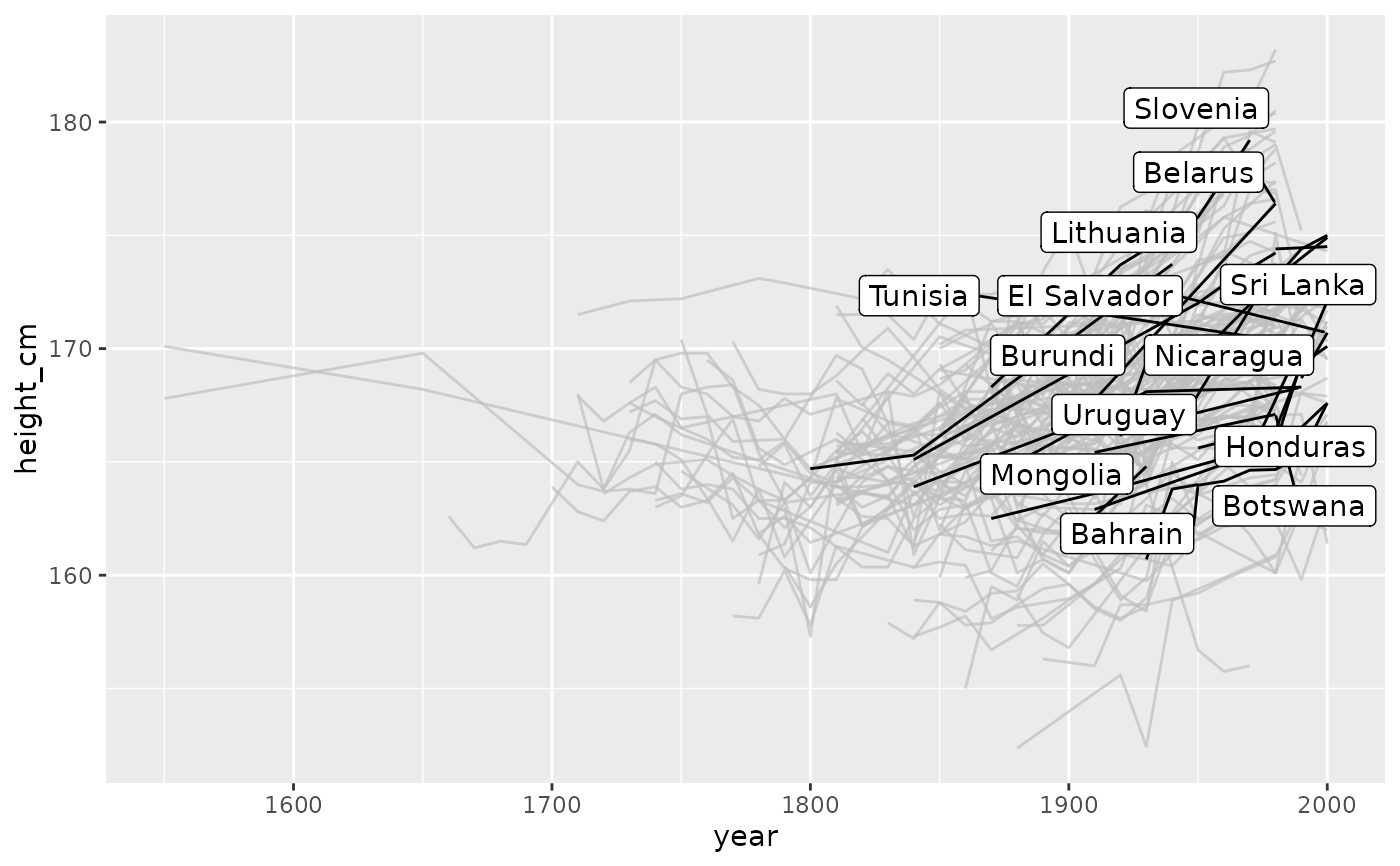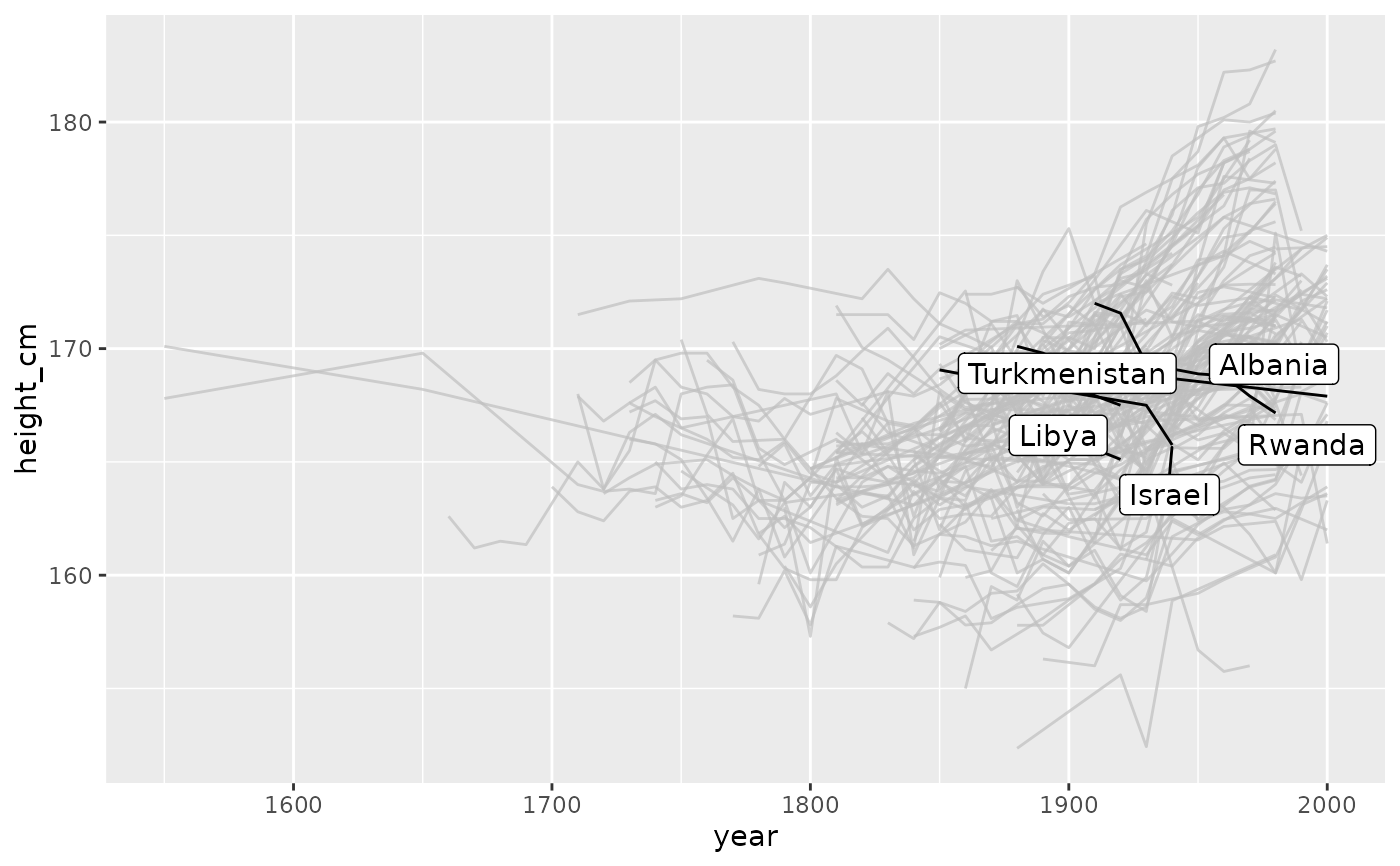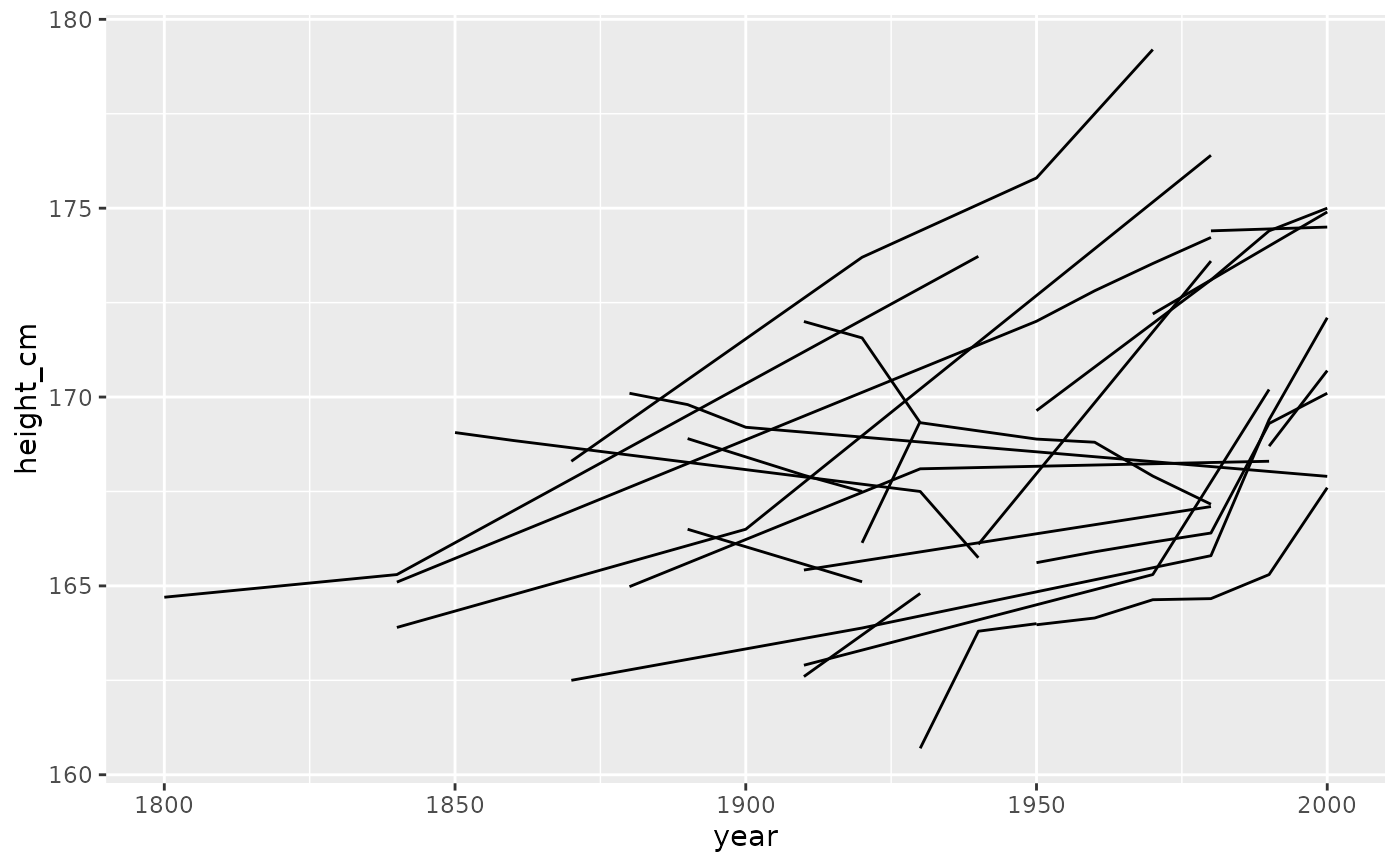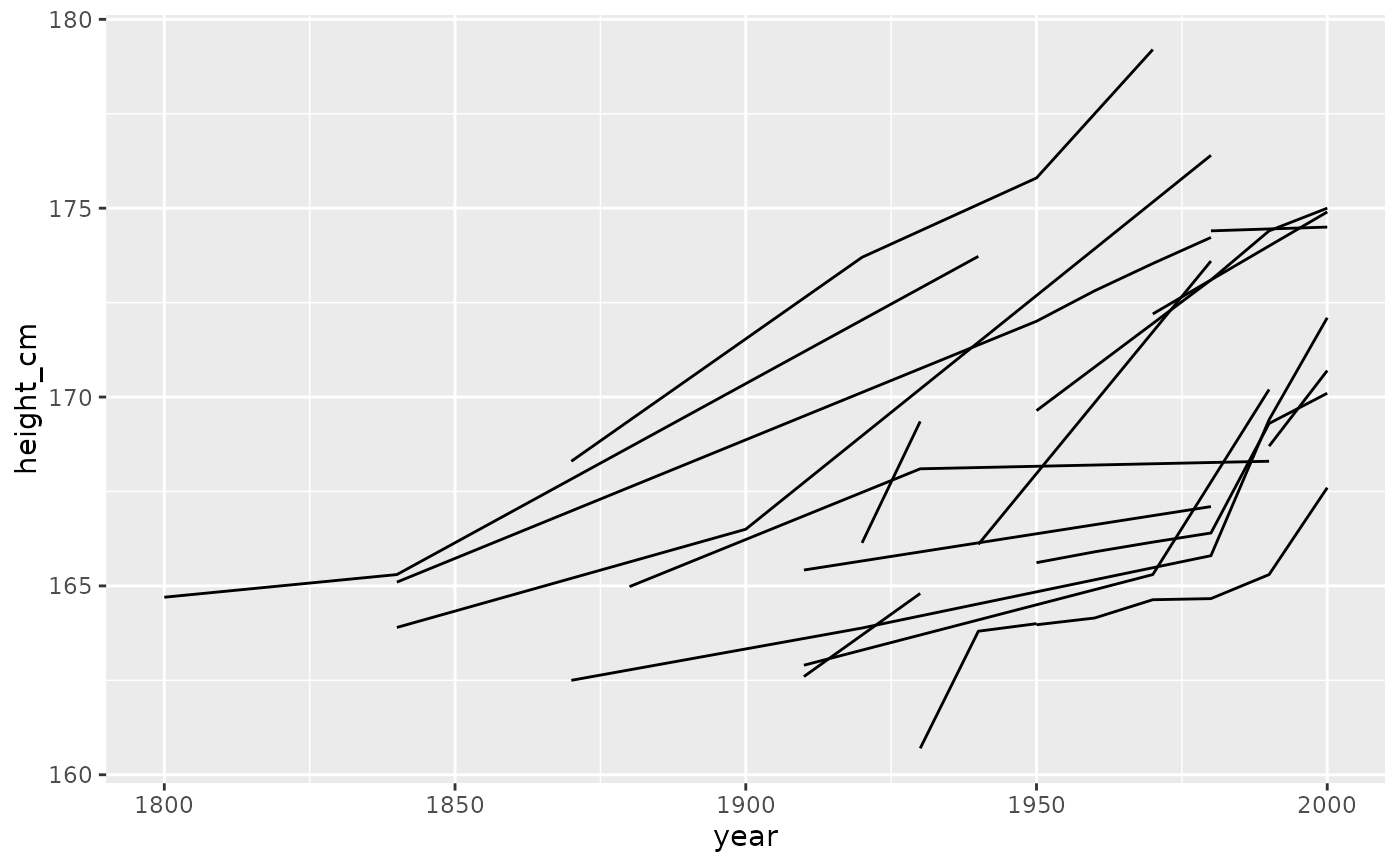These provides three families of functions to tell you if values are always increasing, decreasing, or unvarying, with the functions, increasing(), decreasing(), or unvarying(). Under the hood it uses diff to find differences, so if you like you can pass extra arguments to diff.

increasing(x, ...)

decreasing(x, ...)

unvarying(x, ...)

monotonic(x, ...)

## Arguments

x numeric or integer extra arguments to pass to diff

## Value

logical TRUE or FALSE

## Examples

vec_inc <- c(1:10)
vec_dec<- c(10:1)
vec_ran <- c(sample(1:10))
vec_flat <- rep.int(1,10)

increasing(vec_inc)#>  TRUEincreasing(vec_dec)#>  FALSEincreasing(vec_ran)#>  FALSEincreasing(vec_flat)#>  FALSE
decreasing(vec_inc)#>  FALSEdecreasing(vec_dec)#>  TRUEdecreasing(vec_ran)#>  FALSEdecreasing(vec_flat)#>  FALSE
unvarying(vec_inc)#>  FALSEunvarying(vec_dec)#>  FALSEunvarying(vec_ran)#>  FALSEunvarying(vec_flat)#>  TRUE
library(ggplot2)
library(gghighlight)
library(dplyr)

heights_mono <- heights %>%
features(height_cm, feat_monotonic) %>%
left_join(heights, by = "country")

ggplot(heights_mono,
aes(x = year,
y = height_cm,
group = country)) +
geom_line() +
gghighlight(increase)#> Warning: Tried to calculate with group_by(), but the calculation failed.
#> Falling back to ungrouped filter operation...#> label_key: countryggplot(heights_mono,
aes(x = year,
y = height_cm,
group = country)) +
geom_line() +
gghighlight(decrease)#> Warning: Tried to calculate with group_by(), but the calculation failed.
#> Falling back to ungrouped filter operation...#> label_key: countryheights_mono %>%
filter(monotonic) %>%
ggplot(aes(x = year,
y = height_cm,
group = country)) +
geom_line()heights_mono %>%
filter(increase) %>%
ggplot(aes(x = year,
y = height_cm,
group = country)) +
geom_line()